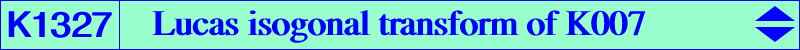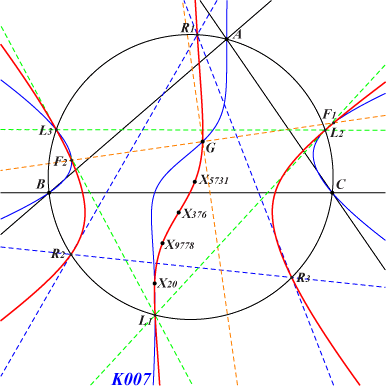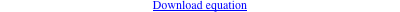too complicated to be written here. Click on the link to download a text file.X(2), X(20), X(376), X(5731), X(9778), X(11206), X(39158), X(39159), X(39160), X(39161) X(54050) → X(54056) vertices of the Lucas triangle L1L2L3, see K007 R1, R2, R3 : antipodes of the vertices of the Thomson triangle, see K002 Geometric properties :K1327 is a central cubic with center X(376) and inflexional tangent at this point parallel to the Brocard axis. K1327 meets the line at infinity at the same points as K004 i.e. the infinite points of the altitudes of ABC. K1327 is actually the image of K004 under the homothety h(X20, 2/3). K1327 is also the image of the analogous cubic K758 under the homothety h(X2, 2). Indeed, K758 is the Thomson isogonal transform of K002. K1327 contains the foci X(39158), X(39159), X(39160), X(39161) of the Steiner circum-ellipse and K758 contains the foci X(39162), X(39163), X(39164), X(39165) of the Steiner in-ellipse.